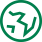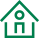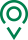• EN
• 圣康首页
• {if condition="\$categorys[\$vo]['is_menu'] == 1"}
• 关于圣康
• {if condition="\$categorys[\$vo]['is_menu'] == 1"} {if condition="\$categorys[\$vo]['is_menu'] == 1"}
• 产品展示
• {if condition="\$categorys[\$vo]['is_menu'] == 1"} {if condition="\$categorys[\$vo]['is_menu'] == 1"}
• 荣誉资质
• {if condition="\$categorys[\$vo]['is_menu'] == 1"} {if condition="\$categorys[\$vo]['is_menu'] == 1"}
• 新闻资讯
• {if condition="\$categorys[\$vo]['is_menu'] == 1"} {if condition="\$categorys[\$vo]['is_menu'] == 1"}
• 联系我们
• {if condition="\$categorys[\$vo]['is_menu'] == 1"} {if condition="\$categorys[\$vo]['is_menu'] == 1"}
• 在线留言
• {if condition="\$categorys[\$vo]['is_menu'] == 1"} {if condition="\$categorys[\$vo]['is_menu'] == 1"}
• 质量管控
• {if condition="\$categorys[\$vo]['is_menu'] == 1"} {if condition="\$categorys[\$vo]['is_menu'] == 1"}
• 源头种植
• {if condition="\$categorys[\$vo]['is_menu'] == 1"} {if condition="\$categorys[\$vo]['is_menu'] == 1"}
• 过程控制
• {if condition="\$categorys[\$vo]['is_menu'] == 1"}

大葱价疲软 洋葱价坚挺

据了解，目前鞍山本地大葱已经陆续上市，由于受到干旱影响,今年本地大葱的品质照往年相比有所下降，价格自然也降了下来。本地大葱价格下降，外地运抵鞍山的洋葱价格则相对稳定。

8月31日，鞍山宁远农产品批发市场商贩在给大葱打捆，由于价格看跌商贩略显惆怅；而在一户批发洋葱的档口里，由于洋葱的批发价格比较喜人，商贩的脸上洋溢着笑容。

据了解，目前鞍山本地大葱已经陆续上市，由于受到干旱影响,今年本地大葱的品质照往年相比有所下降，价格自然也降了下来。据商贩介绍，去年每斤大葱的批发价在1.4元左右，而目前批发价在0.7元左右；本地大葱价格下降，外地运抵鞍山的洋葱价格则相对稳定。

我们的宗旨

•全球精选

一站式购物方案

•多仓直发

特有次日达配送服务

•源头直采

精选正宗好食材

•天天底价

新鲜到家购不停